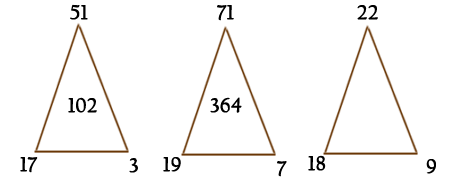## Numbers Puzzles

25. 5 Friends live in the same road A, B, C, D, E.The numbers of B, C, D when multiplied together equals 1260. The numbers B, C, D when added equal twice E’s number, and is even.A’s number is half as much again as E’s. The road numbers run from 2 to 222.What are the 5 house numbers?
Answer
 A 36B 4 C 9 D 35 E 24.
26. Which number goes in the center of the third triangle?Answer
 36 The answer in the centre of each triangle equals the difference between the top and left hand values, multiplied by the right hand value.